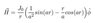# Ampere's law to find free current in a wire

• Benlaww
In summary, the post says that you can find the enclosed current with the help of the azimuthal symmetry of H and the fact that it is in the ##\hat\phi## direction.f

#### Benlaww

Homework Statement
An long straight wire (can be assumed infinite) with a circular cross-section of radius R is lying along the z axis and it has
an internal H~ field given by:

H = (j_0/r) ((1/a^2)sin(ar)-(r/a)cos(ar)) φˆ

where r is the radial distance from the centre of the circular conductor, φˆ is the azimuthal unit
vector, J0 is a constant current density, and a=π/(2R).

Find an expression for the total free current
in the conductor, and give its direction.
Relevant Equations
Ampere's law
∫ H.dl = ∫ j_f .dS = I_f
∇ x H = j_f
I'm not sure how to actually solve this?

Do the integral. What is ##d\vec S##?
H = j_0/r ((1/a^2)sin(ar)-(r/a)cos(ar)) φˆ is dimensionally incorrect. Parameter a apparently has dimensions of inverse length. You can't have (1/a^2)sin(ar)-(r/a)cos(ar). Please fix it.

Last edited:
I know that I have to do the integral but it's actually doing the integral which I'm struggling with. This is the equation that I was given.

#### Attachments

•H.JPG
2.9 KB · Views: 77
You can see that the equation is dimensionally incorrect. You have to ask the person who expects you to solve the problem about it, but let's set it aside for the time being. If you know you have to do the integral, you need to assemble its components. First and foremost is ##d\vec S##. How would you write that as a vector? In other words, what is its direction and what is its magnitude?

Last edited:
So dS is the small surface vector which is the area on the end of the wire (πR^2) pointing normally outward in the z direction?

You got the direction right but not the magnitude. See here how to write the magnitude.

so dS = r dr dθ Z
as there's no terms in θ, after canceling terms becomes:

(j_0/a^2) ∫ sin(ar) dr - (j_0/a) ∫ rcos(ar) dr

I think you are doing the hard way by calculating a surface integral. In the OP you write something that inspires for a clever shortcut, $$\oint \mathbf{H}\cdot d\mathbf{l}=I_{\text{free_enclosed}}$$

This integral will be much easier to find since the given H has azimuthal symmetry and is in the ##\hat\phi## direction, so just choose as loop of integration any circle of radius r.

•kuruman and Steve4Physics
So would it be
∫ H . 2πr dr with bounds 0 and R?

So would it be
∫ H . 2πr dr with bounds 0 and R?
Nope, dl of post #8 and your dr are not the same, the integral of H around a circle of radius r, will essentially be $$H\cdot 2\pi r$$ that is just a multiplication because H has azimuthal symmetry and is in the ##\hat\phi## direction.

So H.2πr = I _ free enclosed? Does r stay as r or does it go to R?

So H.2πr = I _ free enclosed? Does r stay as r or does it go to R?
After you multiply you can put r=R to find the total enclosed current.

Thank you so much! This is a great help!

•Delta2